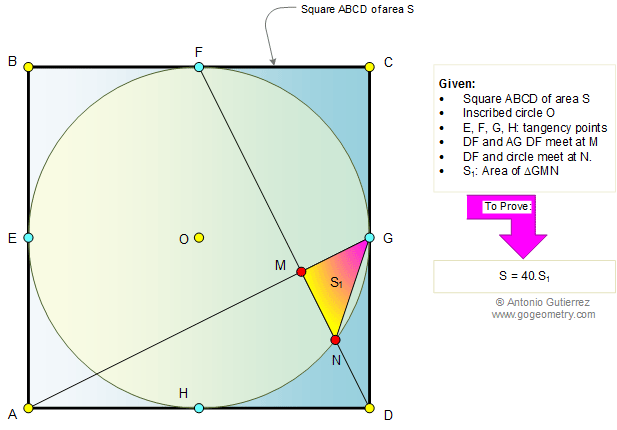Problem 371: Square, Inscribed circle, Triangle, AreaThe figure shows a circle O inscribed in a square ABCD of area S. Points E, F, G, and H are the tangency points. M is the point of intersection of DF and AG and N is de point of intersection of DF and circle O. If S1 is the area of triangle GMN, prove that S = 40S1See also: Typography of problem 371.
 Home | Search | Geometry | Problems | All Problems | 371-380 | Circle Inscribed in a Square | Area of a Triangle | Email | View or post a solution